×#### Thank you for registering.

One of our academic counsellors will contact you within 1 working day.

Click to Chat

1800-1023-196

+91-120-4616500

CART 0

• 0

MY CART (5)

Use Coupon: CART20 and get 20% off on all online Study Material

ITEM
DETAILS
MRP
DISCOUNT
FINAL PRICE
Total Price: Rs.

There are no items in this cart.
Continue Shopping• Complete JEE Main/Advanced Course and Test Series
• OFFERED PRICE: Rs. 15,900
• View Details

```Revision Notes on Mensuration

Plane Figures

The closed 2-D shapes are referred to as plane figures.Here “C” is the boundary of the above figure and the area inside the boundary is the region of this figure. Point D comes in the area of the given figure.

Perimeter

If we go around the figure along its boundary to form a closed figure then the distance covered is the perimeter of that figure. Hence the Perimeter refers to the length of the boundary of a closed figure.

If a figure is made up of line segments only then we can find its perimeter by adding the length of all the sides of the given figure.

Example

Find the Perimeter of the given figure.Solution

Perimeter = Sum of all the sides

= (12 + 3 + 7 + 6 + 10 + 3 + 15 + 12) m

= 68 m

The Perimeter of a Rectangle

A rectangle is a closed figure with two pairs of equal opposite sides.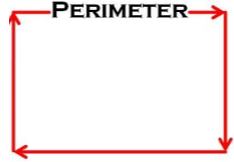Perimeter of a rectangle = Sum of all sides

Thus, Perimeter of a rectangle = 2 × (length + breadth)

Example: 1

The length and breadth of a rectangular swimming pool are 16 and 12 meters respectively .find the perimeter of the pool.

Solution:

Perimeter of a rectangle = 2 × (length + breadth)

Perimeter of the pool = 2 × (16 + 12)

= 2 × 28

= 56 meters

Example: 2

Find the cost of fencing a rectangular farm of length 24 meters and breadth 18 meters at 8/- per meter.

Solution:

Perimeter of a rectangle = 2 × (length + breadth)

Perimeter of the farm = 2 × (24 + 18)

= 2 × 42

= 84 meter

Cost of fencing = 84 × 8

= Rs. 672

Thus the cost of fencing the farm is Rs. 672/-.

Regular Closed Figure

Figures with equal length of sides and an equal measure of angles are known as Regular Closed Figures or Regular Polygon.

Perimeter of Regular Polygon = Number of sides × Length of one side

Perimeter of Square

Square is a regular polygon with 4 equal sides.Perimeter of square = side + side + side + side

Thus, Perimeter of a square = 4 × length of a side

Example

Find the perimeter of a square having side length 25 cm.

Solution

Perimeter of a square = 4 × length of a side

Perimeter of square = 4 × 25

= 100 cm

Perimeter of an Equilateral Triangle

An equilateral triangle is a regular polygon with three equal sides and angles.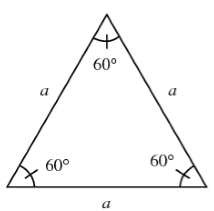Perimeter of an equilateral triangle = 3 × length of a side

Example

Find the perimeter of a triangle having each side length 13 cm.

Solution

Perimeter of an equilateral triangle = 3 × length of a side

Perimeter of triangle = 3 × 13

= 39 cm

Perimeter of a Regular Pentagon

A regular pentagon is a polygon with 5 equal sides and angles.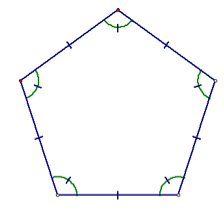Perimeter of a regular pentagon = 5 × length of one side

Example

Find the perimeter of a pentagon having side length 9 cm.

Solution

Perimeter of a regular pentagon = 5 × length of one side

Perimeter of a regular pentagon = 5 × 9

= 45 cm

Perimeter of a Regular Hexagon

A regular hexagon is a polygon with 6 equal sides and angles.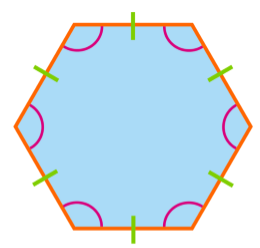Perimeter of a regular hexagon = 6 × Length of one side

Example

Find the perimeter of a hexagon having side length 15cm.

Solution

Perimeter of a regular hexagon = 6 × Length of one side

Perimeter of a regular hexagon = 6 × 15

= 90 cm

Perimeter of a Regular Octagon

A regular octagon is a polygon with 8 equal sides and angles.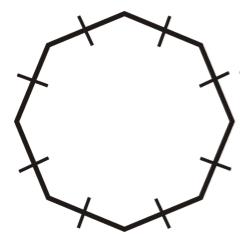Perimeter of a regular octagon = 8 × length of one side

Example

Find the perimeter of an octagon having side length 7cm.

Solution

Perimeter of a regular octagon = 8 × length of one side

Perimeter of a regular octagon = 8 × 7

= 56 cm

Area

Area refers to the surface enclosed by a closed figure.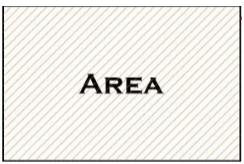To find the area of any irregular closed figure, we can put them on a graph paper with the square of 1 cm × 1 cm .then estimate the area of that figure by counting the area of the squares covered by the figure.

Here one square is taken as 1 sq.unit.

Example

Find the area of the given figure. (1 square = 1 m2)Solution

The given figure is made up of line segments and is covered with some full squares and some half squares.

Full squares in figure = 32

Half squares in figure = 21

Area covered by full squares = 32 × 1 sq. unit = 32 sq. unit.

Area covered by half squares = 21 × (1/2) sq. unit. = 10.5 sq. unit.

Total area covered by figure = 32 + 10.5 = 42.5 sq. unit.

Area of a Rectangle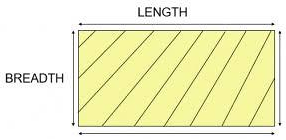Area of a rectangle = (length × breadth)

Example

Find the area of a rectangle whose length and breadth are 20 cm and 12 cm respectively.

Solution

Length of the rectangle = 20 cm

Breadth of the rectangle = 12 cm

Area of the rectangle = length × breadth

= 20 cm × 12 cm

= 240 sq cm.

To find the length of a rectangle if breadth and area are given: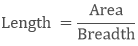Example

What will be the length of the rectangle if its breadth is 6 m and the area is 48sq.m?

SolutionLength = 48/6

= 8 m

To find the breadth of the rectangle if length and area are given: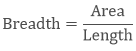Example

What will be the breadth of the rectangle if its length is 8 m and the area is 81 sq.m?

Solution= 9 m

Area of a Square

Area of a square is the region covered by the boundary of a square.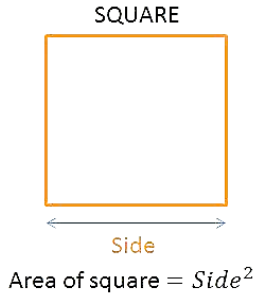Area of a square = side × side

Example

Calculate the area of a square of side 13 cm.

Solution

Area of a square = side × side

= 13 × 13

= 169 cm2.
```### Course Features

• 728 Video Lectures
• Revision Notes
• Previous Year Papers
• Mind Map
• Study Planner
• NCERT Solutions
• Discussion Forum
• Test paper with Video Solution